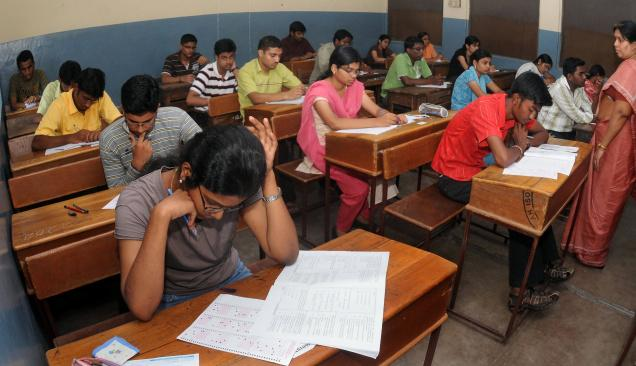# JEE-MainsIn India, there is an exam JEE-Mains which is taken by more than a million students. Here, there are 90 objective problems, each having 4 choices, out of which only 1 is correct. Each correct answer carries 4 marks, and each incorrect answer costs -1 marks. You get 0 marks for an unattempted problem.

Consider a very special student sitting in the exam. This student knows at least 1 of the problems correctly. The probability that he knows the correct answers to exactly $k$ problems is directly proportional to $\frac{1}{k}$. In each of the problems he doesn't know the answer to, there is an 80% chance that he marks it randomly and a 20% chance that he leaves it unanswered.

Find the greatest integer less than or equal to the expected marks of this student.

Details and assumptions

• $\displaystyle \sum_{n=1}^{90} \frac{1}{n} = \frac{1}{0.196751}.$

• If he answers a problem randomly, the probability that he gets it correct is exactly $\frac{1}{4}.$

• As any other student, if he knows the correct answer to a problem, he will mark it, i.e. he won't leave it unanswered.

×